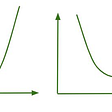# Batch Gradient Descent and Linear Regression

For this blog, I will break down the batch gradient descent and its application to linear regression. Gradient descent is an optimization technique in machine learning and batch means that all observations will be accounted for as a whole.

## Problem:

In short, I have some feature x(univariate) to determine a dependant variable y. A line can be drawn by the following equation.

The x value is the input variable, m is the slope, and b is the y-intercept. The objective is to find the values of m and b in which ŷ(predicted) values are closest to y(actual). The error is calculated by ŷ(predicted) minus y(actual) and since I will be doing gradient descent on all observations, I will sum the squared error.

The RSS here is synonymous with the loss or cost function. I look to minimize RSS by altering m and b simultaneously. I will alter the values of m and b by subtracting the partial derivatives of each term multiplied by some fixed learning rate.

The partial derivative is the derivative of a variable while others are held constant. In other words, it‘s a piece by piece derivative. The partial derivative of m would be the derivative of m given that b is constant.

As the error values may be larger due to having more observations, it’s common to eliminate the 2 multipliers and even divide by the number of observations. I will end up multiplying the partial derivative by a constant learning rate so reducing the derivative value is negligent.

Luckily for us, the RSS function for linear regression is bowl-shaped and the local minima are also the global minima. The m and b values are adjusted simultaneously until the loss has been minimized.

--

--

--

## More from Albert Um

Hello! My name is Albert Um.

Love podcasts or audiobooks? Learn on the go with our new app.

## Student Findings Could Guide Vision-Based Detection Research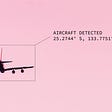## Develop and publish Text Generating Twitter bot## Natural language processing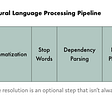## Deploying deep learning models: Part 1 an overview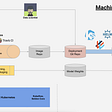## RepNet: Counting Repeating Actions in a Video## Introducing the world of Machine Learning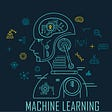## Albert Um

Hello! My name is Albert Um.

## Logistic Regression Breakdown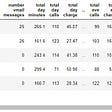## Introduction To Linear Regression